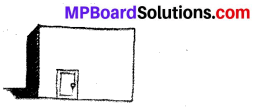# MP Board Class 7th Maths Solutions Chapter 11 Perimeter and Area Ex 11.1

## MP Board Class 7th Maths Solutions Chapter 11 Perimeter and Area Ex 11.1

Question 1.
The length and the breadth of a rectangular piece of land are 500 m and 300 m respectively. Find
(i) its area
(ii) the cost of the land, if 1 m2 of the land costs ₹ 10,000.
Solution:
Length (l) = 500 m
(i) Area = Length × Breadth = 500 × 300 = 150000 m2
(ii) Cost of 1 m2 land = ₹ 10000
∴ Cost of 150000 m2 land
= 150000 × 10000 = ₹ 1500000000

Question 2.
Find the area of a square park whose perimeter is 320 m.
Solution:
Perimeter of the square park = 320 m
∴ 4 × Length of the side of park = 320
Length of the side of park = $$\frac{320}{4}$$ = 80 m
Area = (Length of the side of park)2
= (80)2 = 6400 m2

Question 3.
Find the breadth of a rectangular plot of land, if its area is 440 m2 and the length is 22 m. Also find its perimeter.
Solution:
Area of a rectangular plot = 440 m2
Length = 22 m
Area = Length x Breadth = 440 m2
∴ 22 × Breadth = 440
⇒ Breadth = $$\frac{440}{22}$$ = 20 m
∴ Perimeter = 2 (Length + Breadth)
= 2 (22 + 20) = 2(42) = 84 mQuestion 4.
The perimeter of a rectangular sheet is 100 cm. If the length is 35 cm, find its breadth. Also find the area.
Solution:
Length = 35 cm
Perimeter = 100 cm
∴ 2 (35 + Breadth) = 100
⇒ 35 + Breadth = 50
⇒ Breadth = 50 – 35 = 15 cm
∴ Area = Length × Breadth
= 35 × 15 = 525 cm2

Question 5.
The area of a square park is the same as of a rectangular park. If the side of the square park is 60 m and the length of the rectangular park is 90 m, find the breadth of the rectangular park.
Solution:
Side of the square park = 60 m
Length of the rectangular park = 90 m
Area of the square park = (side)2 = (60)2 = 3600 m2
Area of rectangular park = Length × Breadth
It is given that area of square park = area of rectangular park
∴ 3600 = 90 × Breadth

Question 6.
A wire is in the shape of a rectangle. Its length is 40 cm and breadth is 22 cm. If the same wire is rebent in the shape of a square, what will be the measure of each side. Also find which shape encloses more area?
Solution:
Length of rectangle = 40 cm
Breadth of rectangle = 22 cm
Perimeter of rectangle = Perimeter of square
∴ 2 (Length + Breadth) = 4 × Side of square
⇒ 2 (40 + 22) = 4 × Side of square
⇒ 2 × 62 = 4 × Side of square
∴ Side of square = $$\frac{124}{4}$$ = 31 cm
Now, area of rectangle = 40 × 22 = 880 cm2
Area of square = (Side)2 = 31 × 31 = 961 cm2
As 961 > 880.
Therefore, the square-shaped wire encloses more area than rectangle – shaped wire.Question 7.
The perimeter of a rectangle is 130 cm. If the breadth of the rectangle is 30 cm, find its length. Also find the area of the rectangle.
Solution:
Perimeter = 130 cm
∴ 2 (Length + 30) = 130
⇒ Length + 30 = 65
⇒ Length = 65 – 30 = 35 cm
Now, area = Length × Breadth
= 35 × 30 = 1050 cm2

Question 8.
A door of length 2 m and breadth 1 m is fitted in a wall. The length of the wall is 4.5 m and the breadth is 3.6 m (see the given figure). Find the cost of white washing the wall, if the rate of white washing the wall is ₹ 20 per m2.Solution:
Length of wall = 4.5 m
Breadth of wall = 3.6 m
Area of wall = Length × Breadth
= 4.5 × 3.6
= 16.2 m2
Area of door = 2 × 1 = 2 m2
Area to be white-washed
= Area of wall – Area of door
= 16.2 – 2 = 14.2 m2
Cost of white-washing 1 m2 area = ₹ 20 2.
∴ Cost of white-washing 14.2 m2 area
= 14.2 × 20 = ₹ 284